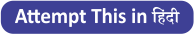Latest Banking jobs   »   Reasoning Ability, Series Quiz For SBI...

# Reasoning Ability, Series Quiz For SBI PO, Clerk Prelims 2021- 15th June

Directions (1–5): Following questions are based on the five three-digit numbers given below:

369    717     922        625      434

Q1. If all the numbers are arranged in ascending order from left to right, which of the following will be the product of the first and last digits of the number which is in the middle in the new arrangements?
(a) 16
(b) 30
(c) 27
(d) 49
(e) 18

Q2. If the positions of the first and third digits of each of the number interchange, what will be the sum of all the digits of the second lowest number?
(a) 13
(b) 15
(c) 16
(d) 11
(e) 20

Q3. If one is subtracted from each odd digit and two is added to each even digit of each of the numbers, what will be the difference between the third digit and the first digit of the lowest number?
(a) Zero
(b) 6
(c) 4
(d) 2
(e) 5

Q4. What will be the resultant if the first digit of the highest number is divided by the third digit of the lowest number?
(a) 0.5
(b) 1
(c) 2
(d) 2.2
(e) None of these

Q5. If all the digits in each of the numbers are arranged in descending order within the number from left to right, which of the following will be the second highest number in the new arrangement
(a) 369
(b) 717
(c) 922
(d) 625
(e) None of these

Directions (6-10): These questions are based on the following alphabet series.

AAFBBFUUABFFAUCBBBFFFCCCCACAUUAABCCUFFCAUCCC

Q6. Which of the following letter is 8th to the right of 15th from right end?
(a) F
(b) U
(c) C
(d) A
(e) B

Q7. How many F’s are there in above arrangement which is immediately followed by vowel?
(a) Three
(b) Two
(c) One
(d) Five
(e) Four

Q8. If all the F’s are deleted from the above arrangement, then which of the following letter is 12th to right of 3rd from left end?
(a) B
(b) U
(c) C
(d) A
(e) None of these

Q9. Which of the following letter is 4th to the left of 11th from left end ?
(a) F
(b) U
(c) C
(d) A
(e) B

Q10. How many B’s are there in above arrangement which is immediately preceded by vowel but not immediately followed by consonant?
(a) Two
(b) One
(c) None
(d) Three
(e) More than three

Direction (11-15): Study the following arrangement carefully and answer the questions given below:

6 4 2 5 2 8 5 2 6 4 1 3 9 1 8 1 2 5 8 6 3 5 1 4 9 4 7 3 2 7 2 5 9

Q11. How many 4s are there in the above arrangement, each of which is immediately preceded by a digit which has a numerical value of more than four?
(a) None
(b) One
(c) Two
(d) Three
(e) More than three

Q12. Which of the following is seventh to the left of the twentieth from the left end of the above arrangement?
(a) 3
(b) 9
(c) 2
(d) 7
(e) 1

Q13. If all the even digits are deleted from the above arrangements, which of the following will be tenth from the right end of the arrangement?
(a) 9
(b) 5
(c) 1
(d) 3
(e) 7

Q14. How many such 5s are there in the above arrangement each of which is immediately preceded by an odd digit and immediately followed by an even digit?
(a) None
(b) One
(c) Two
(d) Three
(e) More than three

Q15. How many such 1s are there in the above arrangement, each of which is immediately preceded by a perfect square?
(a) None
(b) One
(c) Two
(d) Three
(e) More than three

Practice More Questions of Reasoning for Competitive Exams:

###### SBI Clerk Study Plan 2021

SolutionsSolutions (1-5):
S1. Ans.(b)
Sol. exactly middle- 625 after arranging in the ascending order
Product of the first and the last digit of 625= 6×5= 30

S2. Ans.(d)
Sol.434 is the second lowest number after arranging the first and last digit of each of the numbers
Sum of all digits= 4 + 3 + 4 = 11

S3. Ans.(b)
Sol.288 is the lowest one
Required difference = 8 – 2 = 6

S4. Ans.(b)
Sol.
first digit of the highest number = 9
Third digit of the lowest number = 9
So 9/9 = 1

S5. Ans.(c)
Sol. 922 is the second highest number after arranging the digits in descending order.

Solutions (6-10):
S6. Ans (a)

S7. Ans.(b)

S8. Ans.(c)
Sol. 12th to right of 3rd from left= 15th from left= C

S9. Ans.(b)

S10. Ans.(c)
Sol. None

Solutions (11-15):

S11. Ans.(d)
Sol. 64, 64, 94

S12. Ans.(b)
Sol. 7th to left of 20th from left= 20-7=13th from left= 9

S13. Ans.(b)
Sol. 5

S14. Ans.(a)
Sol. None

S15. Ans.(c)
Sol. 41, 91